# AP Physics B : Harmonic Motion

## Example Questions

### Example Question #1 : Ap Physics 1

A spring is compressed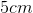from equilibrium by a force of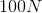. What is the spring constant for this spring?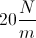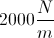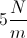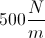Explanation:

We use Hooke's law equation to relate the force, displacement, and spring constant: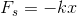We are given the force and the displacement, allowing us to solve for the spring constant.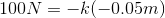Note that the displacement is negative, since the spring is compressed. For springs, compressions represents a negative displacement, while stretching represents a positive displacement.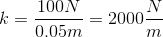### Example Question #1 : Using Spring Equations

A mass of weight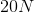is suspended vertically from a spring and stretches it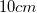from equilibrium. What is the energy stored in the spring?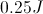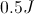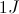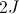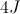Explanation:

First, we need to solve for the spring constant by using the force on the spring. We can use Hooke's Law: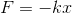The magnitude of the force on the spring will be equal to the force of gravity on the mass, which is given to be. The distance the spring it stretched is in the downward direction, so we must use a negative displacement. Use these values to calculate the spring constant.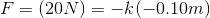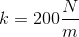Next, use the spring energy equation with the displacement and spring constant to solve for the energy stored in the spring.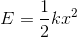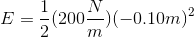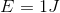### Example Question #1 : Other Potential Energy

A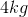block is sliding on a horizontal frictionless floor at a speed of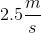and runs into a horizontal spring. The spring has a spring constant of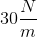. What is the maximum compression of the spring after the collision?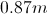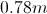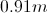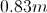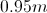Explanation:

First, we calculate the kinetic energy of the block as it slides: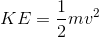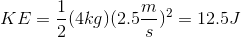We know that all of the kinetic energy is converted to spring potential energy during the collision. We can use the equation for the potential energy of a spring and set it equal to the kinetic energy. Then, solve for the compression distance: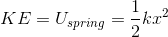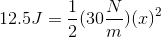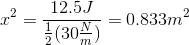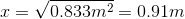### All AP Physics B Resources